# Test: Bearing Capacity & Shallow Foundation

## 10 Questions MCQ Test Topicwise Question Bank for Civil Engineering | Test: Bearing Capacity & Shallow Foundation

Description
Attempt Test: Bearing Capacity & Shallow Foundation | 10 questions in 30 minutes | Mock test for Civil Engineering (CE) preparation | Free important questions MCQ to study Topicwise Question Bank for Civil Engineering for Civil Engineering (CE) Exam | Download free PDF with solutions
QUESTION: 1

### The net ultimate bearing capacity of a purely cohesive soil

Solution:

As per Terzaghi's bearing capacity equation,
qult = cNc + γDf Nq + 0.5 γBNγ
For a purely cohesive soil,
Nq = 1 and Nγ = 0
∴ qult = cNc + γDf
But qnet,ult = qult - γDf
⇒ qnet,ult = cNc + γDf - γFf ⇒ qnet,ult = cNc

QUESTION: 2

### Consider the following statements: increasing width of footing results in 1. Increase in settlement of a consolidating clay layer 2. Increase in bearing capacity of sandy soils 3. Decrease in bearing capacity of clays Which of these statements is/are correct?

Solution:

Changing the width of footing does not affect the bearing capacity of clays.

QUESTION: 3

### If an SPT test gave the average blow count of 32 in fine sand below water table, then what is the corrected value of blow count?

Solution:

Corrected SPT value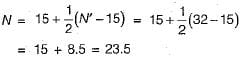QUESTION: 4

Which factors influence the bearing capacity of a purely cohesionless soil?
1. Relative density of soil
2. Width and depth of footing
3. Unit weight of soil
Select the correct answer using the codes given below:

Solution:
QUESTION: 5

Match List-I (Types of foundation) with List-ll (Suitability) and select the correct answer using the codes given below the lists:
List-I
B. Under reamed piles
C. Raft foundation
D. Deep foundation
List-ll
1. Soft clay for 20 m followed by hard rock stratum
2. Up to 3 m black cotton soil followed by medium dense sand
3. Compact sand deposit extending to great depth
4. Loose sand extending to great depth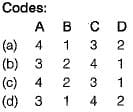Solution:

In the case of loose sand there may be loose pockets causing differential settlements. Raft foundations reduce the differential settlements and higher, allowable soil pressure may be used for design of rafts on loose sands.
Under-reamed piles are suitable to bear the swelling pressure caused by black cotton soil.

QUESTION: 6

A test plate 30 cm square, settles by 12 mm under a load of 4.5 kN in a sandy soil. By how much will a footing 2 m x 2 m subjected to a load of 200 kN settle?

Solution:

Load intensity for 4.5 kN load on 30 cm square plate will be,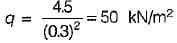Load intensity for 200 kN load on 2 m x 2 m footing will be,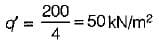Since load intensity is same so,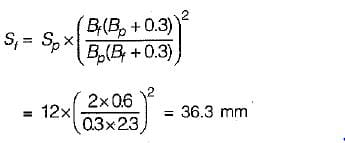QUESTION: 7

In which one of the following zones is a logarithmic spiral shape of failure surface assumed in the case of bearing capacity analysis of c-ϕ soils?

Solution:

According to Terzaghi there are three zones: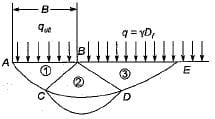Zone-1: Elastic zone of wedge shape located beneath the loaded strip wherein the major principal stress are vertical. This remains in elastic equilibrium and behaves as if it were a part of footing itself and ∠CAB = ∠CBA = ϕ.
Zone-2: Radial shear zone where arc CD is logarithmic spiral. (Circular when ϕ = 0).
Zone-3: It is called Rankine passive zone. An overburden pressure q = γDf acts as a surcharge on this zone.

QUESTION: 8

The bearing capacity factors Nc, Nq and Nare functions of

Solution:

Terzaghi’s bearing capacity factors Nc, Nq and Nγ are function of angle of friction only.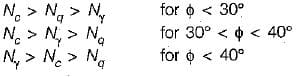QUESTION: 9

When a load test was conducted by putting a 60 cm square plate on top of a sandy deposit, the ultimate bearing capacity was observed as 60 kN/m2. What is the ultimate bearing capacity for a strip footing of 1.2 m width to be placed on the surface of the same soil?

Solution:

According to Terzaghi,
qnu = 0.5 γB Nγ for strip footing
= 0.4 γB Nγ for square footing Using the above equations,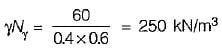For strip footing,
qnu = 0.5 x 1.2 x 250 = 150 kN/m2

QUESTION: 10

The ultimate bearing capacity of a square footing on surface of a saturated clay having unconfined compression strength of 50 kN/m2 (using Skempton’s equation) is

Solution:

qnu = cuNc
where cu = 50/2 = 25 kN/m2
Nc = 6(1 + 0.2 Df/B)
for Df = 0, we get Nc = 6
∴ qnu = 25 x 6 = 150 kN/m2Use Code STAYHOME200 and get INR 200 additional OFF Use Coupon Code